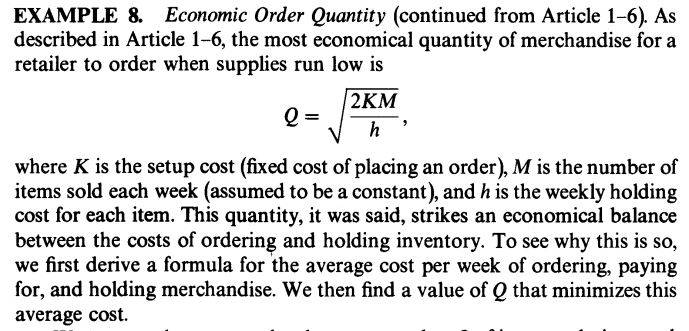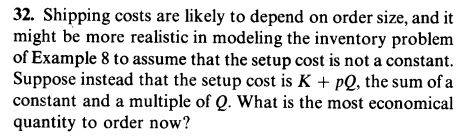# Minimization problem: Economics: quantity to order

• Karol

## Homework Statement## Homework Equations

Minimum/Maximum occurs when the first derivative=0

## The Attempt at a Solution

$$Q=\sqrt{\frac{2(K+pQ)}{h}}~\rightarrow~Q=\frac{2}{h}(KM+pM)$$
##Q'=0~## gives no sense result

Karol said:

## Homework Statement

View attachment 230767
View attachment 230768

## Homework Equations

Minimum/Maximum occurs when the first derivative=0

## The Attempt at a Solution

$$Q=\sqrt{\frac{2(K+pQ)}{h}}~\rightarrow~Q=\frac{2}{h}(KM+pM)$$
##Q'=0~## gives no sense result

You cannot just write ##Q = \sqrt{2(K+pQ)/h}##. You must start the whole modelling procedure over again.

In the old model, the average cost per week was given as
$$C(Q) = \frac{KM}{Q} + \frac{1}{2} h Q \; \hspace{2cm} (1)$$
Solving ##C'(Q)=0## gave the economic order quantity as ##Q = \sqrt{2KM/h}.##

What is the equation that replaces (1) in the new model?

Last edited:
In the old model:
$$A(Q)=\frac{KM}{Q}+cM+\frac{hQ}{2}$$
Where c is the purchase cost of one item. in the new model:
$$A(Q)=\frac{K+pQ}{Q/M}+cM+\frac{hQ}{2}=\frac{KM}{Q}+(c+p)M+\frac{hQ}{2}$$
And differentiating gives the same result

Karol said:
In the old model:
$$A(Q)=\frac{KM}{Q}+cM+\frac{hQ}{2}$$
Where c is the purchase cost of one item. in the new model:
$$A(Q)=\frac{K+pQ}{Q/M}+cM+\frac{hQ}{2}=\frac{KM}{Q}+(c+p)M+\frac{hQ}{2}$$
And differentiating gives the same result

Correct.

BTW: why do you call this thread a "min max" problem? It is a "minimization" problem, but there is no "max" involved. In mathematics, operations research, economics and other such fields the term "min-max" has a definite meaning, and it is nothing like how you use it.

Last edited:
Thank you Ray, you are correct, this is only a minimum problem.
I just automatically wrote the heading.
Thanks